# Additional Questions Answers: Polynomials Notes | Study Mathematics (Maths) Class 10 - Class 10

## Document Description: Additional Questions Answers: Polynomials for Class 10 2022 is part of Mathematics (Maths) Class 10 preparation. The notes and questions for Additional Questions Answers: Polynomials have been prepared according to the Class 10 exam syllabus. Information about Additional Questions Answers: Polynomials covers topics like and Additional Questions Answers: Polynomials Example, for Class 10 2022 Exam. Find important definitions, questions, notes, meanings, examples, exercises and tests below for Additional Questions Answers: Polynomials.

Introduction of Additional Questions Answers: Polynomials in English is available as part of our Mathematics (Maths) Class 10 for Class 10 & Additional Questions Answers: Polynomials in Hindi for Mathematics (Maths) Class 10 course. Download more important topics related with notes, lectures and mock test series for Class 10 Exam by signing up for free. Class 10: Additional Questions Answers: Polynomials Notes | Study Mathematics (Maths) Class 10 - Class 10
 1 Crore+ students have signed up on EduRev. Have you?

Q 1. How many zeroes can the polynomial 2x- 3x + 4 have?

It is a quadratic polynomial i.e. the degree of 2x- 3x + 4 is 2.
∴ It can have two zeroes at the most.

Q 2. The graph of y = p (x) is shown in the figure below. How many zeroes does p (x) have?

Since the curve (graph) of p (x) is intersecting the x-axis at three points,
∴ y = p (x) has 3 zeroes.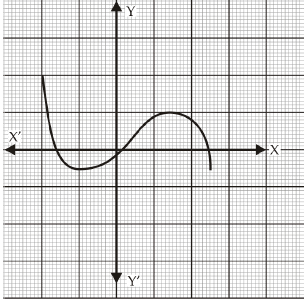Q 3. What is the value of p (x) = x2 - 3x - 4 at x = 2?

Given,
p(x) = x2 - 3x - 4
⇒ p(2) = (2)2 - 3 (2) - 4
⇒ 4 - 6 - 4 = - 6

Q 4. What is the value of p (x) = x2 - 3x - 4 at x = - 1?

We have,
p(x) = x- 3x - 4
⇒ p (- 1) = (- 1)- {3 (- 1)} - 4
⇒ 1 + 3 - 4 = 0

Q 5. What is the zero of 2x + 3?

Since,
The zero of a linear polynomial =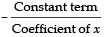∴ The zero of 2x + 3 = -3/2

Q 6. The Coefficient of x and the constant term in a linear polynomial are 5 and - 3 respectively. Find its zero.

Since,
The zero of a linear polynomial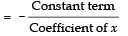∴ The zero of the given linear polynomial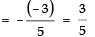Q 7. The graph of a quadratic polynomial x2 - 3x - 4 is a parabola. Determine the opening of the parabola.

In x2 - 3x - 4, the Coefficient of x2 is 1 and 1 > 0
∴ The parabola opens upwards.

Q 8. Find the sum of the zeroes of the polynomial 2x- 8x + 6.
p (x) = x2 - 3x - 4

p (x) = 2x2 - 8x + 6
∴ a = 2, b = - 8 and c = 6
⇒ Sum of the zeroes = -b/a
⇒ -(-8)/(2) = 4

Q 9. Find the product of zeroes of the quadratic polynomial 3x2 + 5x - 2.

Here, a = 3, b = 5 and c = - 2
As, Product of the zeroes = c/a
⇒ Product of the zeroes of 3x2 + 5x - 2 = -2/3

Q 10. If a fifth degree polynomial is divided by a quadratic polynomial, write the possible degree of the quotient.

∵ Degree of the dividend p(x) = 5
Degree of the divisor f(x) = 2
∴ Degree of the quotient q(x) = Degree of p(x) - Degree of f(x) = 5 - 2 = 3

Q 11. Graph of a linear polynomial is a straight line. If the points (0, 4), (1, 2) and (2, 0) lie on this graph, write the zero of the polynomial.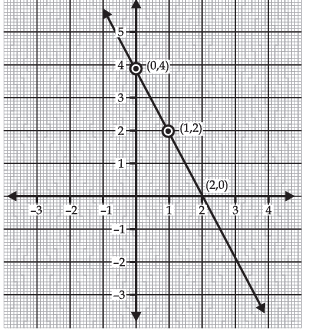y = p (x) has its zero at a point where y = 0
In the figure, y = 0 at (2, 0).
∴ 2 is the zero of p (x).

Q 12. For what value of k, (- 4) is a zero of the polynomial x2 - x - (2k + 2)?

Since, (- 4) is the zero of p (x)
⇒ p (- 4) = (- 4)2 - (- 4) - (2k + 2) = 0
⇒ 16 + 4 = 2k + 2
⇒ 20 - 2 = 2k
⇒ 18= 2k
⇒ k = 18/2 = 9
Thus, the required value of k = 9.

Q 13. For what value of p, (- 4) is a zero of the polynomial x2 - 2x - (7p + 3)?

We have
∵ p (x) = x2 - 2x - (7p + 3)
p (- 4) = (- 4)2 - 2 (- 4) - (7p + 3)
Since - 4 is a zero of p (x),
∴ (- 4)2 - 2 (- 4) - (7p + 3) = 0
⇒ 16 + 8 = 7p + 3
⇒ 24 - 3 = 7p
⇒ 7p = 21
⇒ p = 21/7= 3
Thus, the required value of p is 3.

Q 14. The sum and product of the zeroes of a quadratic polynomial are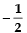and - 3 respectively. What is the quadratic polynomial?

For a quadratic polynomial, we have
Sum of zeroes = -1/2

Product of the zeroes = -3
Since general equation of the quadratic polynomial
= x2 - [Sum of the roots] x + [Product of the roots]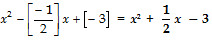Q 15. The graph of y = f (x) is given in the figure, find the number of zeroes of f (x).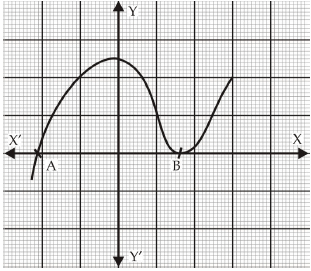From the figure (graph), it is evident that y = f (x) intersects the x-axis at two distinct points A and B.
∴ Number of zeroes of f (x) = 2.

Q 16. In the adjoining figure the graph of a polynomial p (x) is given. Find the zeroes of the polynomial.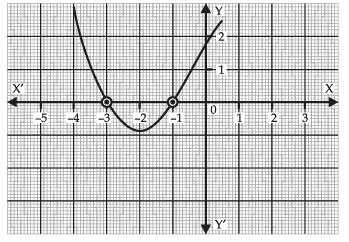From the graph, it is evident that the graph of the given polynomial intersects the x-axis at - 1 and - 3.
∴ The zeroes of the polynomial are - 1 and - 3.

Q 17. If 1 is a zero of the polynomial p (x) = ax2 - 3 (a - 1) x - 1, then find the value of a.

Here, p (x) = ax2 - 3(a - 1)x - 1
∴ p(1) = a(1)- 3(a - 1) × 1 - 1
= a - 3a + 3 - 1
= - 2a + 2
∵ 1 is a zero of p (x);
∴ p (1) = 0
⇒ - 2a + 2 = 0 ⇒ a = 1

Q 18. If (x + a) is a factor of 2x2 + 2ax + 5x + 10 find a.

Here, p(x) = 2x+ 2ax + 5x + 10
∵ (x + a) is a factor of p(x)
∴ - a is a zero of p(x)
⇒ p (- a) = 0
⇒ 2 (- a)2 + 2a (- a) + 5 (- a) + 10 = 0
⇒ 2a2 - 2a2 - 5a + 10 = 0
⇒ a = 10/5 = 2
Thus, the required value of a = 2.

Q 19. Write the zeroes of the polynomial x2 + 2x + 1.

We have,
p (x) = x2 + 2x + 1
= (x + 1)2
If p (x) = 0 then (x + 1)2 = 0
⇒ x = - 1
Thus, the zero of the polynomial p (x) is - 1.

Q 20. Write the zeroes of the polynomial x2 - x - 6.

We have,
p (x) = x2 - x - 6
= x2 - 3x + 2x - 6
⇒ x (x - 3) + 2 (x - 3)
⇒ (x - 3) (x + 2)
For p (x) = 0
⇒ (x - 3) (x + 2) = 0
∴ x = 3 and x = - 2

Q 21. Write a quadratic polynomial, the sum and product of whose zeroes are 3 and - 2 respectively.

A quadratic polynomial is given by
x2 - [Sum of the zeroes] x + [Product of zeroes]
But, Sum of zeroes = 3
Product of zeroes = - 2
∴ The required quadratic polynomial
= x- 3x + (- 2)
= x2 - 3x - 2

Q 22. Write the number of zeroes of the polynomial y = f (x) whose graph is given in the figure.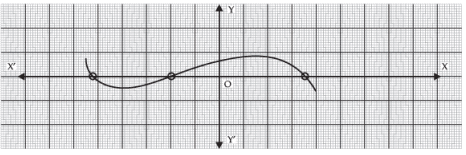Since, curve (graph) of y = f (x) intersects the x-axis at three points,
∴ y = f (x) has three zeroes.

Q 23. The graph of y = f (x) is given in figure. How many zeroes are there of f (x)?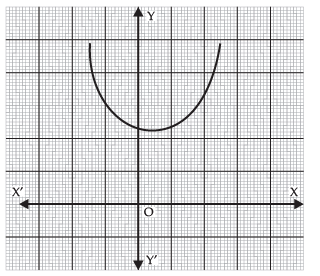The graph of y = f (x) does not intersect the x-axis.
∴ The number of zeroes of f (x) is 0.

Q 24. The graph of y = f (x) is given in the figure. What is the number of zeroes of f (x)?

Since, the graph of y = f (x) intersects the x-axis at three distinct points,
∴ y = f (x) has 3 zeroes.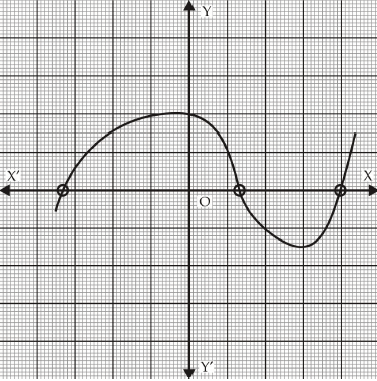Q 25. What is the number of zeroes of the polynomial y = p (x)?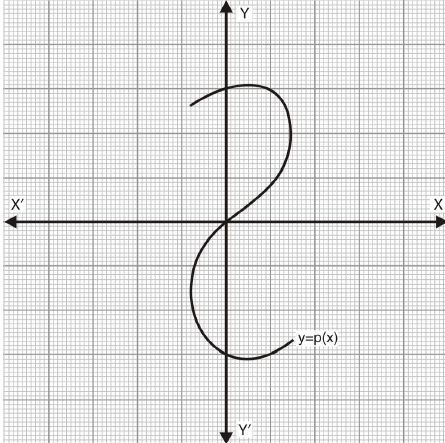Since, the graph passes through origin(0,0) which means on putting x = 0, we get y = 0.
Therefore, (0,0) is the only root of this equation.

The document Additional Questions Answers: Polynomials Notes | Study Mathematics (Maths) Class 10 - Class 10 is a part of the Class 10 Course Mathematics (Maths) Class 10.
All you need of Class 10 at this link: Class 10

## Mathematics (Maths) Class 10

53 videos|369 docs|138 tests
 Use Code STAYHOME200 and get INR 200 additional OFF

## Mathematics (Maths) Class 10

53 videos|369 docs|138 tests

Track your progress, build streaks, highlight & save important lessons and more!

,

,

,

,

,

,

,

,

,

,

,

,

,

,

,

,

,

,

,

,

,

;